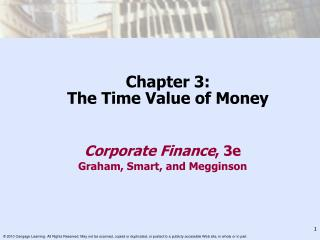DownloadDownload PresentationChapter 3: The Time Value of Money

# Chapter 3: The Time Value of Money

Télécharger la présentation## Chapter 3: The Time Value of Money

- - - - - - - - - - - - - - - - - - - - - - - - - - - E N D - - - - - - - - - - - - - - - - - - - - - - - - - - -
##### Presentation Transcript

1. Chapter 3:The Time Value of Money Corporate Finance, 3e Graham, Smart, and Megginson

2. The Role of Financial Markets • Voluntary transfer of wealth • Between individuals • Financial intermediaries • Across time • Future value • Present value • The chance to earn a return on invested funds means a dollar today is worth more than a dollar in the future.

3. FVn = PV  (1+r)n • Future Value depends on: • Interest Rate • Number of Periods • Compounding Interval Future Value The Value of a Lump Sum or Stream of Cash Payments at a Future Point in Time

4. Future Value • Two key points: • The higher the interest rate, the higher the future value. • The longer the period of time, the higher the future value.

5. Present Value • Compounding: • Finding the future value of present dollars invested at a given rate • Discounting: • Finding the present value of a future amount, assuming an opportunity to earn a given return (r), on the money

6. Present Value Today's Value of a Lump Sum or Stream of Cash Payments Received at a Future Point in Time FVn = PV  (1+r)n PV =

7. FV of a Mixed Stream • The future value of any stream of cash flows measured at the end of a specified year is the sum of the future values of the individual cash flows at that year’s end. • Sometimes called the terminal value

8. FV of a Mixed Stream Equation Future value of an n-year mixed stream of cash flows (FV) can be expressed as

9. FV of Annuities: Formulas • FV of an ordinary annuity: • FV of an annuity due:

10. Present Value Of Perpetuity Stream of Equal Annual Cash Flows that Lasts Forever or

11. Present Value of a Growing Perpetuity The Gordon Growth model:

12. Compounding More Frequentlythan Annually • Semiannually • Quarterly • Monthly • Continuous

13. Compounding Intervals m compounding periods per year

14. For semiannual compounding, m = 2: • For quarterly compounding, m = 4: Compounding More Frequently Than Annually FV at End of 2 Years of \$125,000 Deposited at 5.13% Interest

15. Continuous Compounding • Interest Compounded Continuously FVn = PV ern FV at End of 2 Years of \$125,000 at 5.13% Annual Interest, Compounded Continuously

16. The Stated Rate Versus the Effective Rate Stated Rate – The contractual annual rate (r) charged by lender or promised by borrower Effective Annual Rate (EAR) – The annual rate actually paid or earned

17. Calculating Deposits Needed To Accumulate A Future Sum • Often need to find annual deposit required to accumulate a fixed sum of money in n years • Closely related to the process of finding the future value of an ordinary annuity

18. Loan Amortization • Generalize the formula to more frequent compounding periods by dividing the interest rate by m and multiplying the number of compounding periods by m.

19. Implied Interest or Growth Rates: Lump Sums • Lump sums: The interest or growth rate of a single cash flow over time can be found by solving for r in the following equation: • If we know the interest rate (r), we can calculate the number of periods (n) necessary for a present value to grow to a desired future value

20. Implied Interest or Growth Rates: Lump Sums • Annuities and mixed streams: Very difficult to solve for r using formulas • Use an iterative trial-and-error approach. Spreadsheets and financial calculators can do this very quickly. • Often referred to as finding the yield to maturityor internal rate of return (IRR)

21. Number of Compounding Periods Lump Sums/Annuities: